# Fisseha Berhane, PhD

#### Data Scientist443-970-2353[email protected] CV Resume### Linear and non-linear regression models to predict global temperature

In this post, I used different linear (generalized linear model, ridge-regression, partial least squares, lasso and elastic net) and non-linear (support vector machines, classification and regression trees, random forest, neural network and boosting) regression models to predict global average temperature anomaly. First, I did exploratory data analysis to understand the features and how they can be associated with global temperature then built predicitve models employing various machine learning techniques, assessed the importance of the various independet variables in predicting average global temperature anomaly and compared the performance of the different models considered. The data is from the MITX Analytics Edge edx course Website.

Let's download the data and see the variables in the data set.

In :
# Load required libraries
require(ggplot2)
library(caret)
library(nnet)
library(NeuralNetTools)

In :
# Download data

url<-"https://courses.edx.org/asset-v1:[email protected]+block/climate_change.csv"

str(clim)

'data.frame':	308 obs. of  11 variables:
$Year : int 1983 1983 1983 1983 1983 1983 1983 1983 1984 1984 ...$ Month   : int  5 6 7 8 9 10 11 12 1 2 ...
$MEI : num 2.556 2.167 1.741 1.13 0.428 ...$ CO2     : num  346 346 344 342 340 ...
$CH4 : num 1639 1634 1633 1631 1648 ...$ N2O     : num  304 304 304 304 304 ...
$CFC.11 : num 191 192 193 194 194 ...$ CFC.12  : num  350 352 354 356 357 ...
$TSI : num 1366 1366 1366 1366 1366 ...$ Aerosols: num  0.0863 0.0794 0.0731 0.0673 0.0619 0.0569 0.0524 0.0486 0.0451 0.0416 ...
$Temp : num 0.109 0.118 0.137 0.176 0.149 0.093 0.232 0.078 0.089 0.013 ...  Let's use the data upto 2006 for training and predict for 2007 and 2008. We will not use the variables 'Year' and 'Month' in predicitive model building process. In : training<-subset(clim,clim$Year<=2006)
testing<-subset(clim,clim$Year > 2006) testingX<-subset(clim[,3:10],clim$Year> 2006)
testingY<-subset(clim[,11],clim$Year> 2006)  ### Exploratory Data Analysis¶ In : ggplot(training, aes(Year, Temp))+geom_point(color="purple")+ ggtitle('Fig.1. Temperature anomaly over time')+ xlab('')+ylab('Temperature')+ stat_smooth(method=lm, colour='blue',span=0.2)  Out: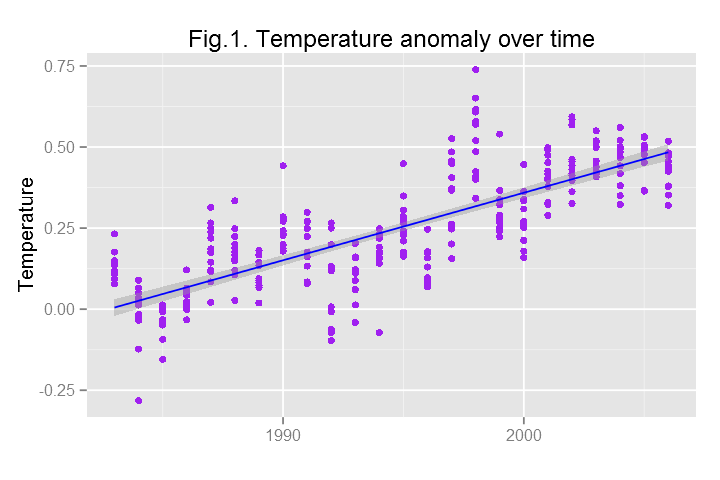From Fig.1., we see the clear increasing trend of temperature over the data period. One of the main causes of global temperature increase is asscoaited with inceased carbondioxide emission to the atmosphere from different sources. Let's see the relationship between temperature and carbondiodixe. In : ggplot(training, aes(CO2, Temp))+geom_point()+ ggtitle('Fig.2. Temperature anomaly vs Carbondioxe concentration')+ xlab(' Carbondioxide')+ylab('Temperature')+stat_smooth(method=lm,colour='blue', span=0.2)  Out: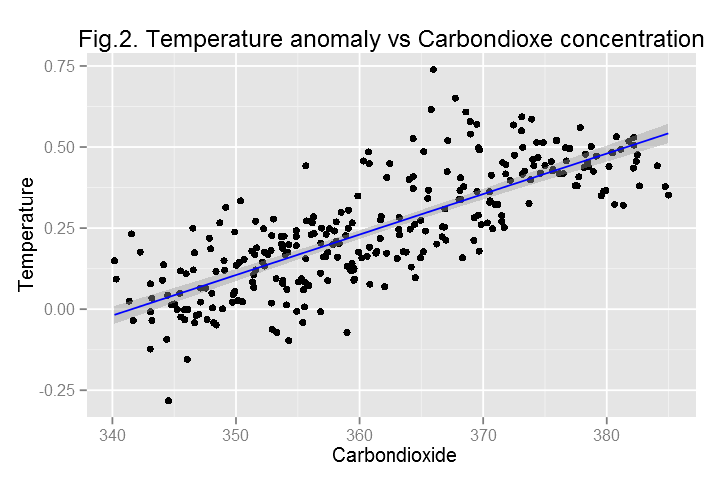As shown in Fig.2, carbondioxide and temperature are strongly related. Following an oscillation in the tropical Pacific Ocean, global tempreature fluctuates. This oscillation is represented by the variable MEI in the dataset. Let's plot the association of temperature anomaly with the MEI index. In : ggplot(training, aes(MEI, Temp))+geom_point()+ ggtitle('Fig.3.Temperature anomaly vs MEI')+xlab(' MEI')+ ylab('Temperature')+stat_smooth(method=lm,colour='blue', span=0.2)  Out: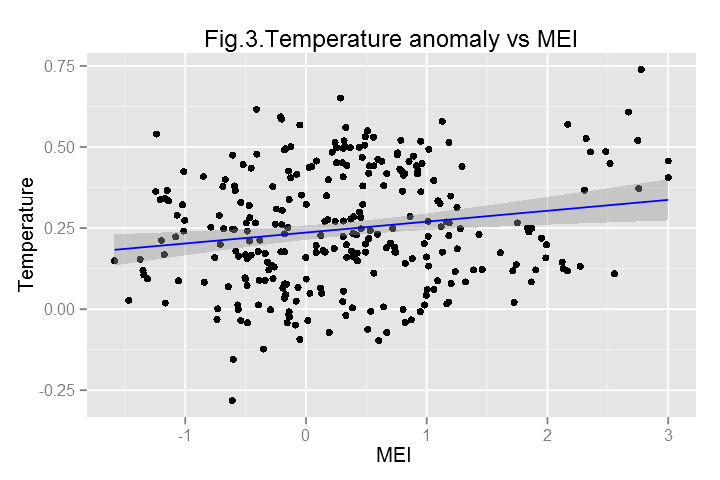The figure does not reveal strong linear association between MEI and average global temperature, however, MEI can have systematic influences on global temperature and it could be an important predictor as we will see later. ## Generalized Linear Model¶ Let's fit a generalized linear model with the "glm" function and generate variable importance figure, predic on the test data and plot observed values versus predicted values using the ggplot2 package. To access accuracy, let's use repeated k-fold cross-validation (particularly 10-fold cross-validation repeated 5 times). In : control <- trainControl(method="repeatedcv", number=10, repeats=5) my_glm <- train(training[,3:10], training[,11], method = "glm", preProc = c("center", "scale"), trControl = control) # Predict using the test data pred<-predict(my_glm,testingX) my_data=as.data.frame(cbind(predicted=pred,observed=testingY)) ggplot(my_data,aes(predicted,observed))+geom_point()+geom_smooth(method=lm)+ggtitle('GLM') # Print, plot variable importance print(varImp(my_glm, scale = FALSE)) plot(varImp(my_glm, scale = FALSE), main="Variable Importance using GLM")  Out: glm variable importance Overall MEI 9.9232 Aerosols 7.2103 TSI 6.3126 CFC.11 4.0778 CFC.12 3.7573 CO2 2.8264 N2O 1.9297 CH4 0.2405  Out: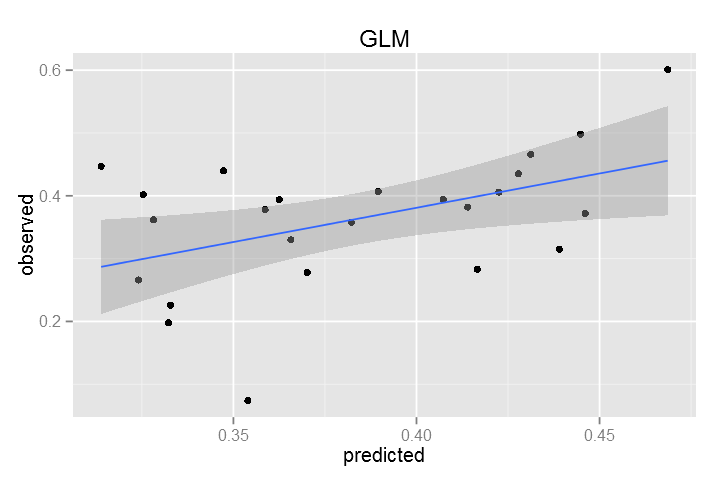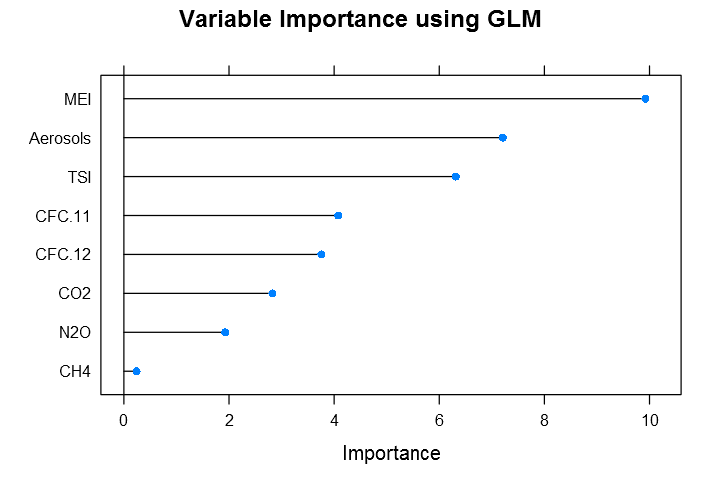We can see the summary of the model and understand which features are significant. In : summary(my_glm)  Out: Call: NULL Deviance Residuals: Min 1Q Median 3Q Max -0.25888 -0.05913 -0.00082 0.05649 0.32433 Coefficients: Estimate Std. Error t value Pr(>|t|) (Intercept) 0.247799 0.005442 45.534 < 2e-16 *** MEI 0.059688 0.006015 9.923 < 2e-16 *** CO2 0.073870 0.026136 2.826 0.00505 ** CH4 0.005665 0.023558 0.240 0.81015 N2O -0.078649 0.040756 -1.930 0.05467 . CFC.11 -0.139159 0.034126 -4.078 5.96e-05 *** CFC.12 0.224856 0.059845 3.757 0.00021 *** TSI 0.037376 0.005921 6.313 1.10e-09 *** Aerosols -0.046150 0.006401 -7.210 5.41e-12 *** --- Signif. codes: 0 '***' 0.001 '**' 0.01 '*' 0.05 '.' 0.1 ' ' 1 (Dispersion parameter for gaussian family taken to be 0.00841098) Null deviance: 9.2853 on 283 degrees of freedom Residual deviance: 2.3130 on 275 degrees of freedom AIC: -540.2 Number of Fisher Scoring iterations: 2  From the summary of the linear model, we see that all features except CH4 are important predictors. ## Generalized Linear Model with Stepwise Feature Selection¶ Now, let's use the glmStepAIC method to select important features automatically via Akaike Information Criterion (AIC). In : control <- trainControl(method="repeatedcv", number=10, repeats=5) my_stepglm <- train(training[,3:10], training[,11], method = "glmStepAIC", preProc = c("center", "scale"), trControl = control) # Predict using the test data pred<-predict(my_stepglm,testingX) my_data=as.data.frame(cbind(predicted=pred,observed=testingY)) ggplot(my_data,aes(predicted,observed))+geom_point()+geom_smooth(method=lm)+ggtitle('GLMStepAIC') # Print, plot variable importance print(varImp(my_stepglm, scale = FALSE)) plot(varImp(my_stepglm, scale = FALSE), main="Variable Importance using GLMStepAIC")  Out: loess r-squared variable importance Overall CFC.12 0.64706 CO2 0.64428 N2O 0.62275 Aerosols 0.57937 CH4 0.54393 CFC.11 0.49591 TSI 0.14404 MEI 0.08436  Out: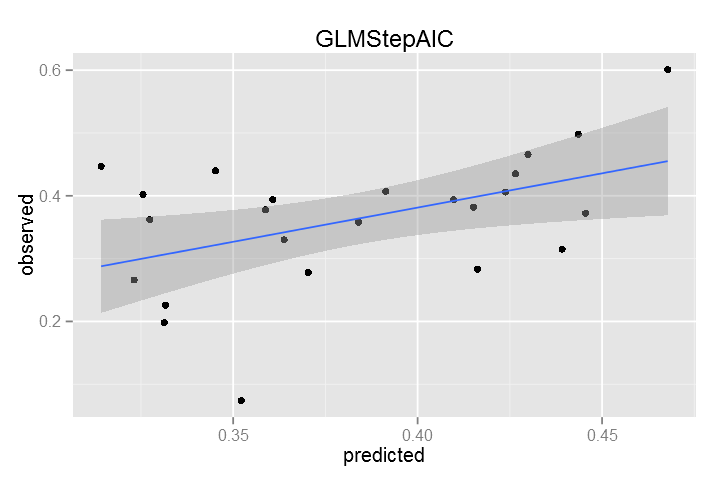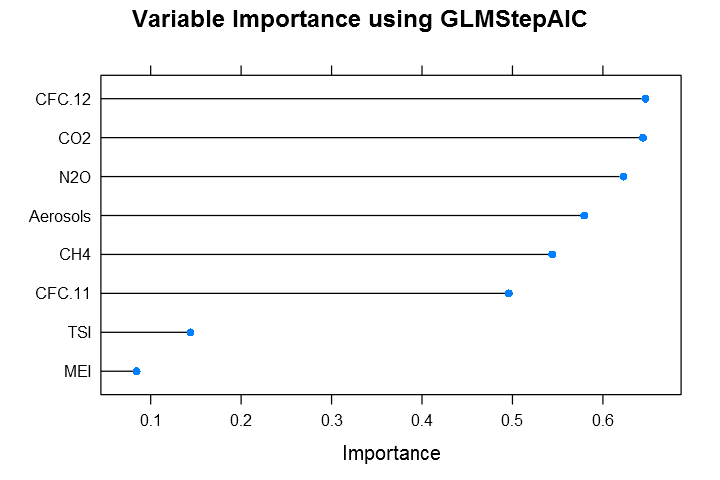## Partial Least Squares (PLS)¶ Prior to performing PLS, the predictors should be centered and scaled, especially if the predictors are on scales of differing magnitude. PLS will seek directions of maximum variation while simultaneously considering correlation with the response. In : control <- trainControl(method="repeatedcv", number=10, repeats=5) my_tunedpls <- train(training[,3:10], training[,11], method = "pls", ## The default tuning grid evaluates ## components 1... tuneLength tuneLength = 20, trControl = control, preProc = c("center", "scale")) # Predict using test data pred<-predict(my_tunedpls,testingX) my_data=as.data.frame(cbind(predicted=pred,observed=testingY)) ggplot(my_data,aes(predicted,observed))+geom_point()+geom_smooth(method=lm)+ggtitle('PLS') # Print, plot variable importance print(varImp(my_tunedpls, scale = FALSE)) plot(varImp(my_tunedpls, scale = FALSE), main="Variable Importance using PLS") summary(my_tunedpls) plot(my_tunedpls)  Out: pls variable importance Overall CO2 0.030823 N2O 0.030341 CFC.12 0.026560 CH4 0.025814 CFC.11 0.021456 Aerosols 0.018157 MEI 0.014342 TSI 0.009778  Out: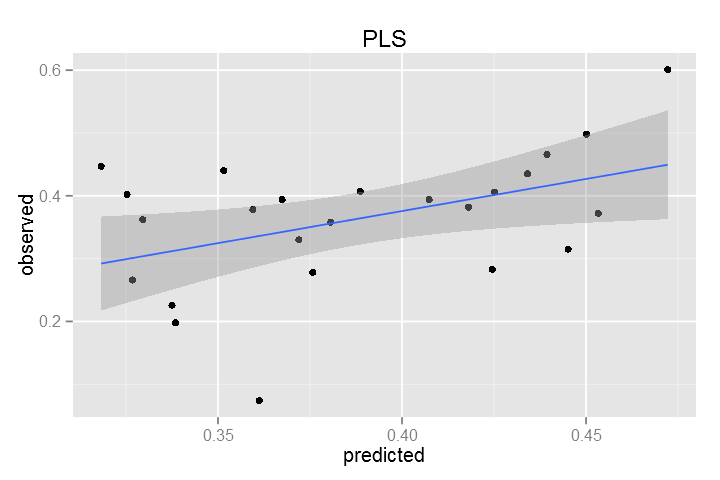Data: X dimension: 284 8 Y dimension: 284 1 Fit method: oscorespls Number of components considered: 7 TRAINING: % variance explained 1 comps 2 comps 3 comps 4 comps 5 comps 6 comps 7 comps X 55.20 64.41 75.94 83.59 96.03 99.46 99.75 .outcome 60.77 73.15 73.86 73.95 74.09 74.49 75.05  Out: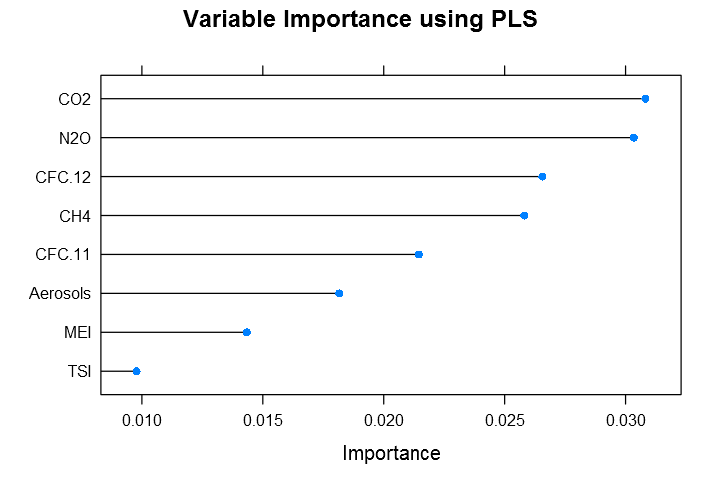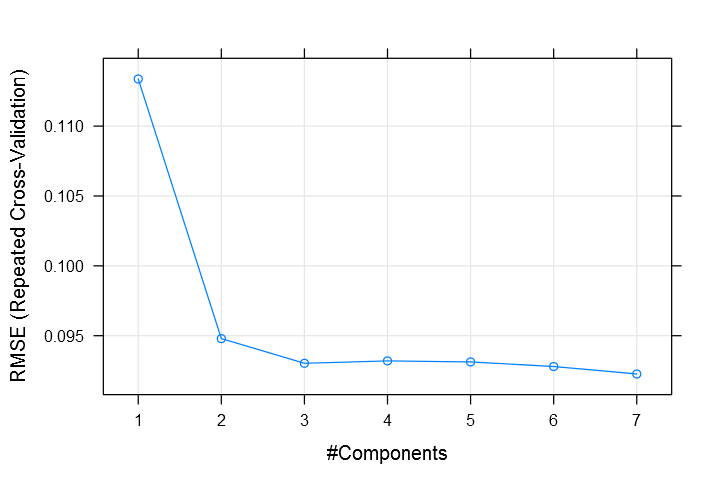## Penalized linear regression models¶ ### 1. Ridge-regression¶ In : ridgeGrid <- data.frame(.lambda = seq(0, .1, length = 15)) control <- trainControl(method="repeatedcv", number=10, repeats=5) set.seed(100) my_ridgeReg <- train(training[,3:10], training[,11], method = "ridge", ## Fit the model over many penalty values tuneGrid = ridgeGrid, trControl = control, ## put the predictors on the same scale preProc = c("center", "scale")) # Predict using the test data pred<-predict(my_ridgeReg,testingX) my_data=as.data.frame(cbind(predicted=pred,observed=testingY)) ggplot(my_data,aes(predicted,observed))+geom_point()+geom_smooth(method=lm)+ggtitle('Ridge Regression') # Print, plot variable importance print(varImp(my_ridgeReg, scale = FALSE)) plot(varImp(my_ridgeReg, scale = FALSE), main="Variable Importance using Ridge Regression") plot(my_ridgeReg)  Out: loess r-squared variable importance Overall CFC.12 0.64706 CO2 0.64428 N2O 0.62275 Aerosols 0.57937 CH4 0.54393 CFC.11 0.49591 TSI 0.14404 MEI 0.08436  Out: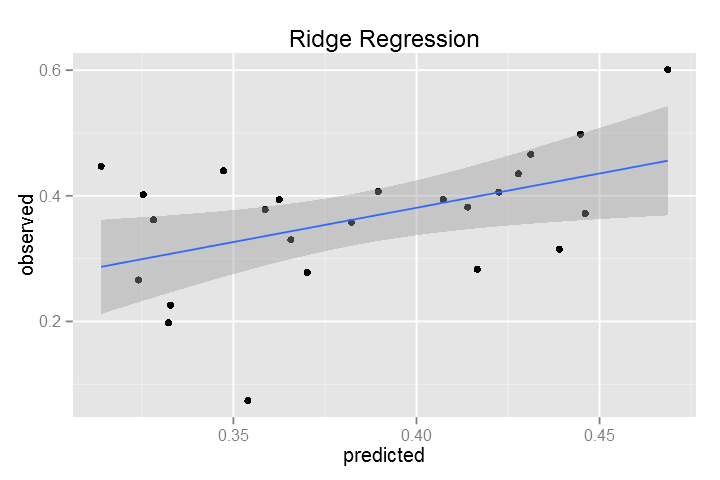Out: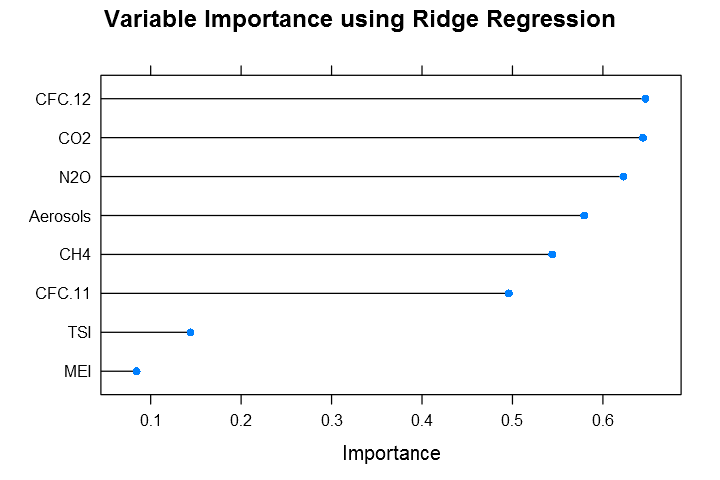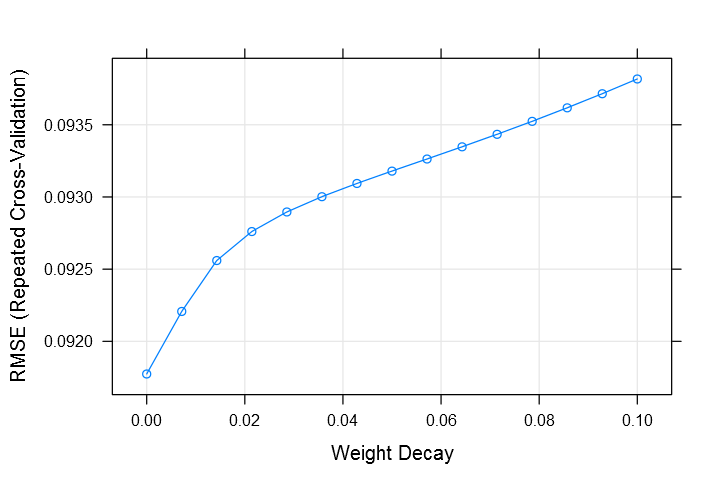### 2. Lasso (least absolute shrinkage and selection operator)¶ In : lassoGrid <- expand.grid(.fraction = seq(.05, 1, length = 20)) control <- trainControl(method="repeatedcv", number=10, repeats=5) set.seed(100) my_lasso <- train(training[,3:10], training[,11], method = "lasso", ## Fit the model over many penalty values tuneGrid = lassoGrid, trControl = control, ## put the predictors on the same scale preProc = c("center", "scale")) # Predict using test data pred<-predict(my_lasso,testingX) my_data=as.data.frame(cbind(predicted=pred,observed=testingY)) ggplot(my_data,aes(predicted,observed))+geom_point()+geom_smooth(method=lm)+ggtitle('lasso') # Print, plot variable importance print(varImp(my_lasso, scale = FALSE)) plot(varImp(my_lasso, scale = FALSE), main="Variable Importance using lasso") plot(my_lasso)  Out: loess r-squared variable importance Overall CFC.12 0.64706 CO2 0.64428 N2O 0.62275 Aerosols 0.57937 CH4 0.54393 CFC.11 0.49591 TSI 0.14404 MEI 0.08436  Out: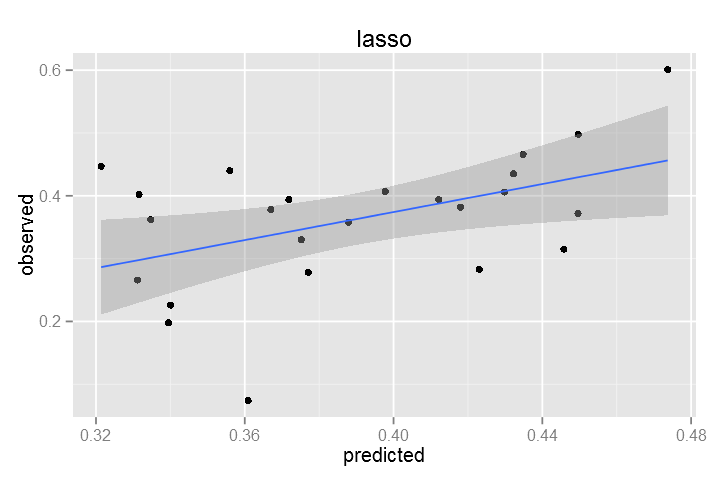Out: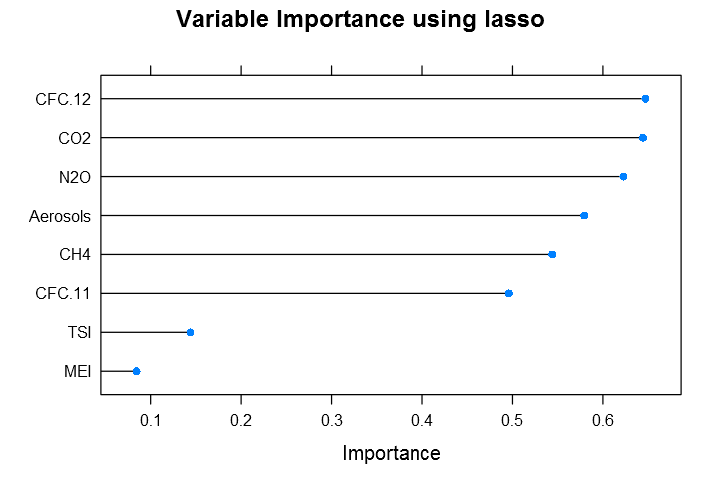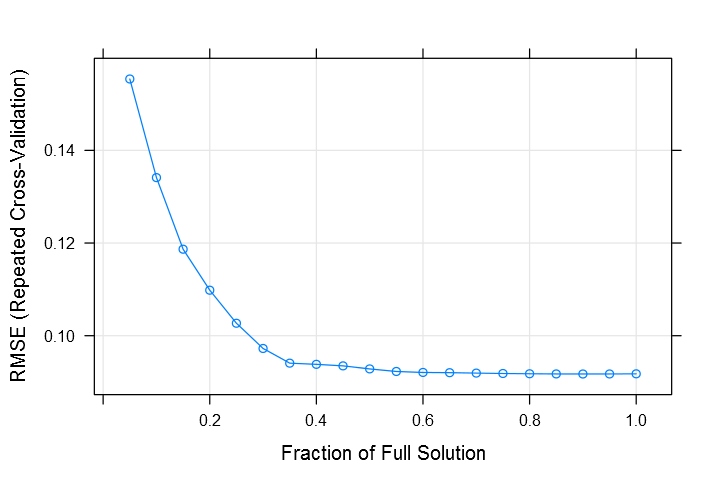### 3. Elastic net¶ In : enetGrid <- expand.grid(.lambda = c(0, 0.01, .1), .fraction = seq(.05, 1, length = 20)) control <- trainControl(method="repeatedcv", number=10, repeats=5) set.seed(100) my_enet <- train(training[,3:10], training[,11], method = "enet", ## Fit the model over many penalty values tuneGrid = enetGrid, trControl = control, ## put the predictors on the same scale preProc = c("center", "scale")) # Predict using the test data pred<-predict(my_enet,testingX) my_data=as.data.frame(cbind(predicted=pred,observed=testingY)) ggplot(my_data,aes(predicted,observed))+geom_point()+geom_smooth(method=lm)+ggtitle('Elastic net') # Print, plot variable importance print(varImp(my_enet, scale = FALSE)) plot(varImp(my_enet, scale = FALSE), main="Variable Importance using elastic net model") plot(my_enet)  Out: loess r-squared variable importance Overall CFC.12 0.64706 CO2 0.64428 N2O 0.62275 Aerosols 0.57937 CH4 0.54393 CFC.11 0.49591 TSI 0.14404 MEI 0.08436  Out: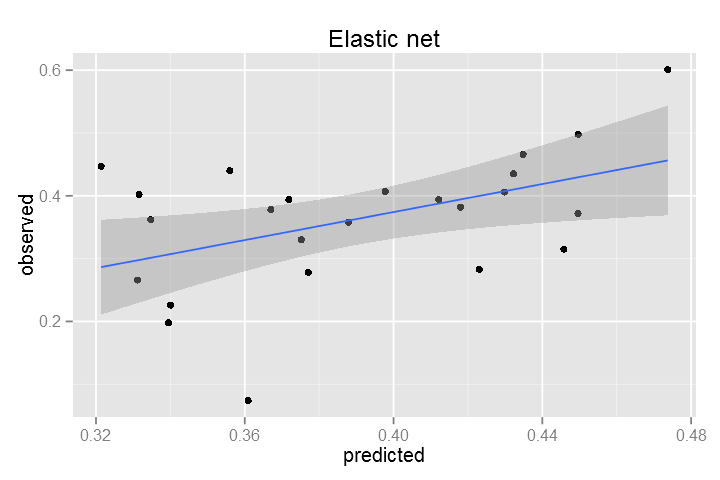Out: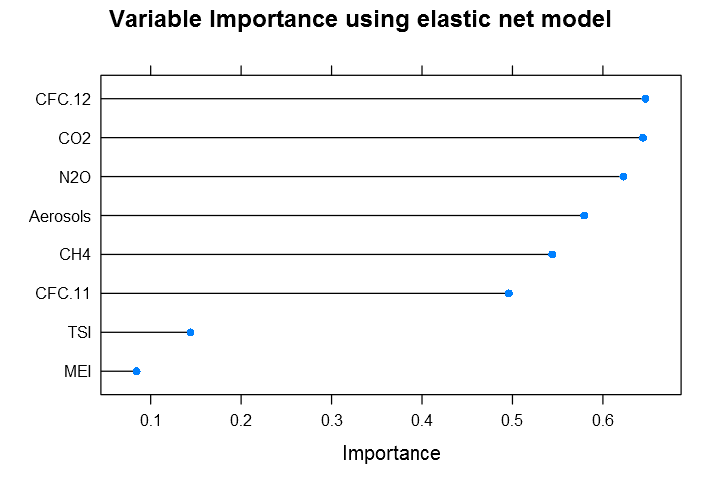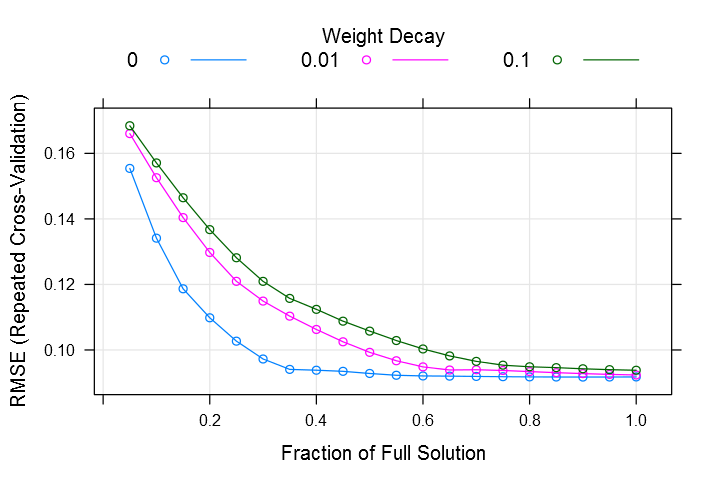## Non-linear regression models¶ Now, let's work with non-linear regression models: neural network, support vector machines, random forest, boosting, and classification and regression trees. ### Neural networks¶ To choose the number of hidden units and the amount of weight decay via resampling, the train function can be applied. First, we remove predictors to ensure that the maximum absolute pairwise correlation between the predictors is less than 0.75. In : # The findCorrelation takes a correlation matrix and determines the # column numbers that should be removed to keep all pair-wise correlations below a threshold tooHigh <- findCorrelation(cor(training[,3:10]), cutoff = .75) trainXnnet <- training[,3:10][, -tooHigh] testXnnet <- testing[,3:10][, -tooHigh] ## Create a specific candidate set of models to evaluate: nnetGrid <- expand.grid(.decay = seq(0, 0.1, .01), .size = c(3:10), ## The next option is to use bagging ## instead of different random ## seeds. .bag = FALSE) set.seed(100) control <- trainControl(method="repeatedcv", number=10, repeats=5) my_avnnet <- train(trainXnnet, training[,11], method = "avNNet",repeats = 10, tuneGrid = nnetGrid, trControl = control, ## Automatically standardize data prior to modeling and prediction preProc = c("center", "scale"), linout = TRUE, trace = FALSE, MaxNWts = 10 * (ncol(trainXnnet) + 1) + 10 + 1, maxit = 500) # Predict using the test data pred<-predict(my_avnnet,testXnnet) my_data=as.data.frame(cbind(predicted=pred,observed=testingY)) ggplot(my_data,aes(predicted,observed))+geom_point()+geom_smooth(method=lm)+ggtitle('ANN') # Print, plot variable importance print(varImp(my_avnnet, scale = FALSE)) plot(varImp(my_avnnet, scale = FALSE), main="Variable Importance using AvNNet")  Out: loess r-squared variable importance Overall CO2 0.64428 Aerosols 0.57937 CFC.11 0.49591 TSI 0.14404 MEI 0.08436  Out: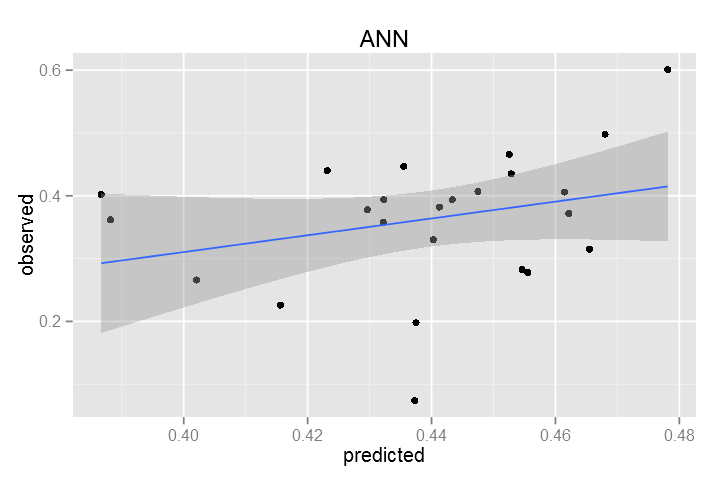Out: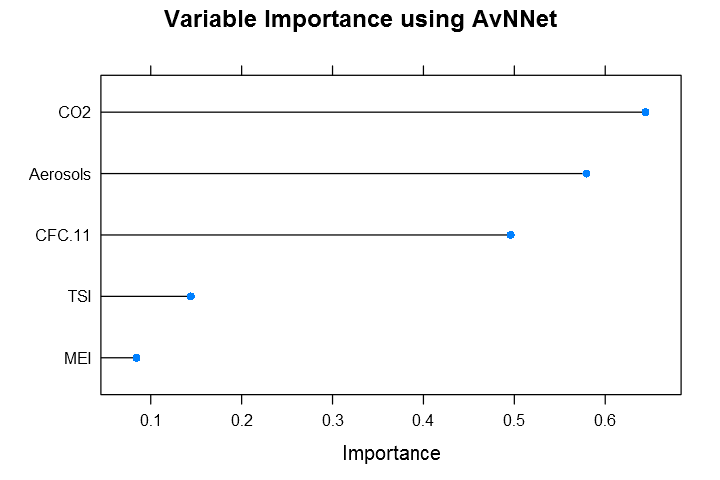Now let's visualize the neural nets used for averagig. In : for (i in 1:length(my_avnnet$finalModel$model)) { allmods <- my_avnnet$finalModel$model mod <- allmods[[i]] # first model, change this number to look at the other models wts <- mod$wts
decay <- mod$decay struct <- mod$n

# recreate
recmod <- nnet(trainXnnet, training[,11], Wts = wts, decay = decay, size = struct, maxit = 0)

# use the functions to look at the individual model

plotnet(recmod)

}

# weights:  36
# weights:  36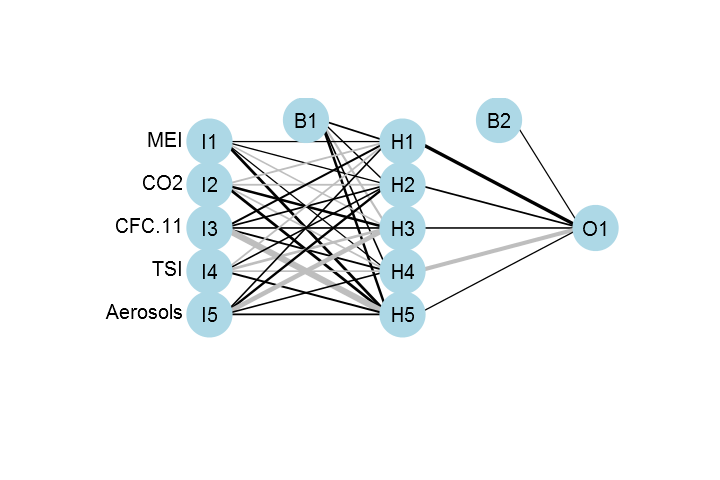# weights:  36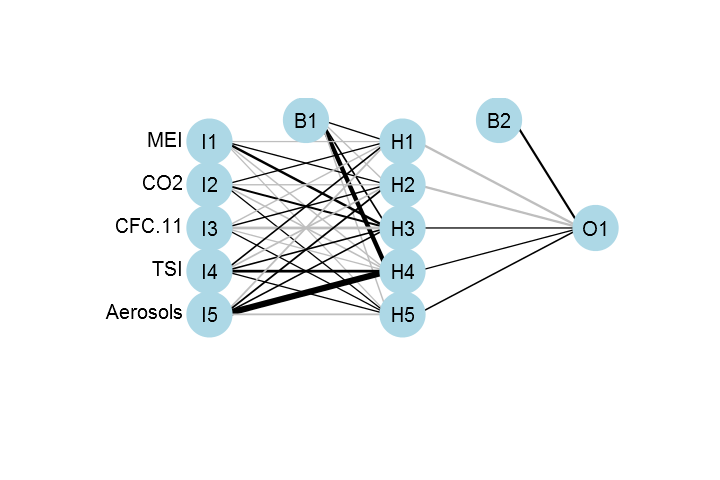# weights:  36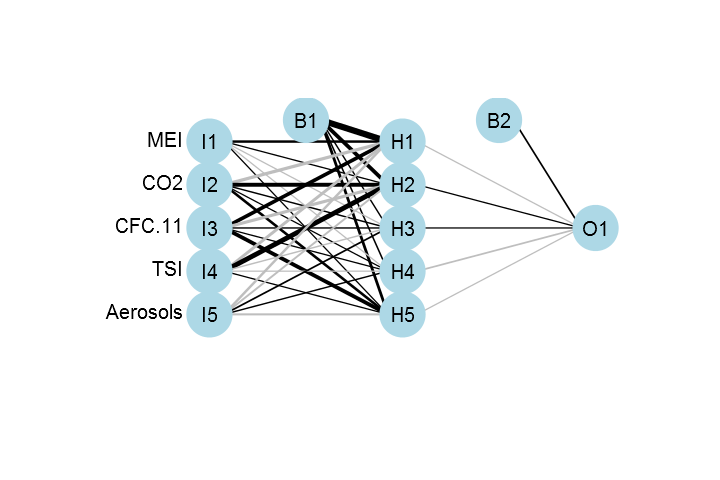# weights:  36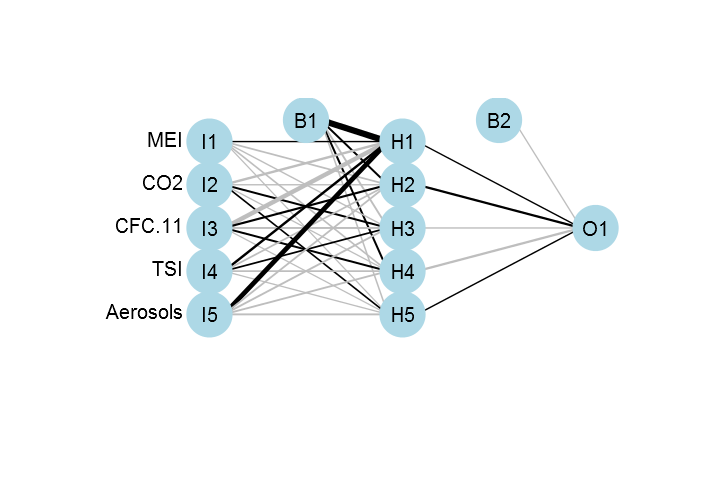# weights:  36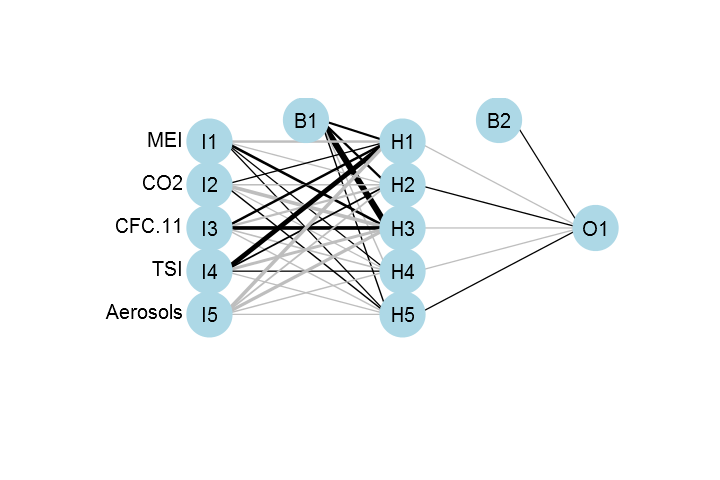# weights:  36